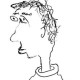A counterexampletotallydisconnected

Let \$latex C/mathbf{Q}_p\$ be a complete algebraically closed nonarchimedean field extension, and let \$latex X\$ be any proper rigid space over \$latex C\$. Let \$latex mathbf{L}\$ be any \$latex mathbf{Z}_p\$-local system on \$latex X_{mathrm{proet}}\$. By the main results in Scholze’s p-adic Hodge theory paper, the pro-etale cohomology groups \$latex H^i_{mathrm{proet}}(X,mathbf{L})\$ are always finitely generated \$latex mathbf{Z}_p\$-modules. It also seems likely that Poincare duality holds in this setting (and maybe someone has proved this?).

Suppose instead that we’re given a \$latex mathbf{Q}_p\$-local system \$latex mathbf{V}\$. By analogy, one might guess that the cohomology groups \$latex H^i_{mathrm{proet}}(X,mathbf{V})\$ are always finitely generated \$latex mathbf{Q}_p\$-vector spaces. Indeed, this (and more) was claimed as a theorem by Kedlaya-Liu in a 2016 preprint. However, it is false. The goal of this post is to work out an explicit counterexample.

So, consider \$latex X=mathbf{P}^1\$ as a rigid space over \$latex C\$. This is the target of the…

View original post 1,124 more words